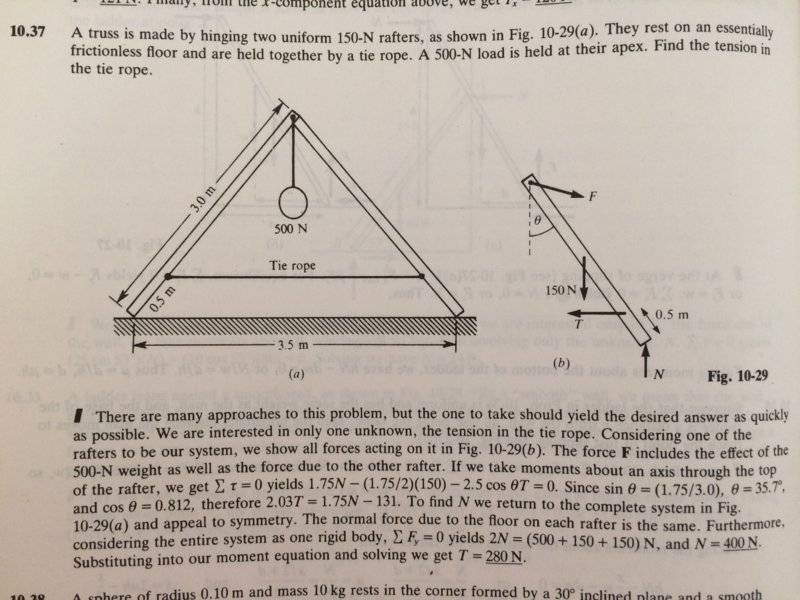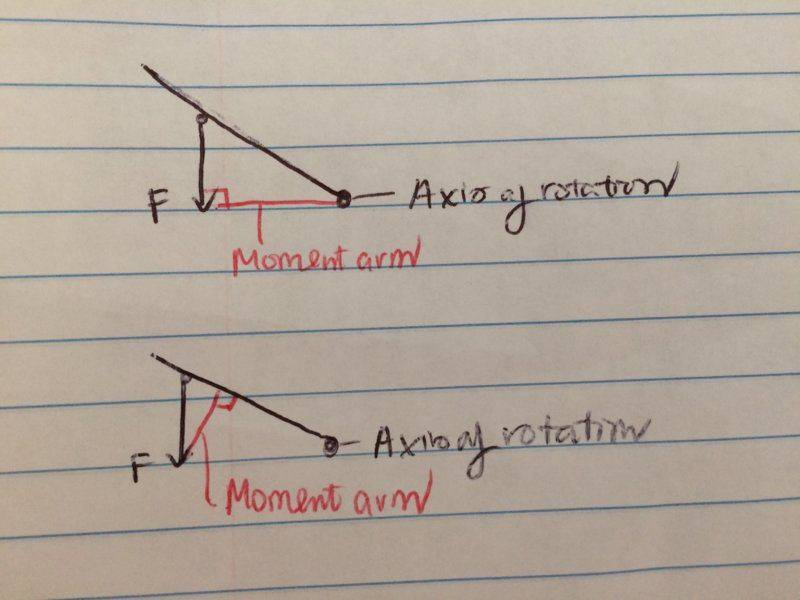# Equilibrium of Rigid Bodies. Rafter. What is moment arm?

## Homework Statement

Two uniform 150 N rafters rest on frictionless floor, held together by a rope. A 500 N load is held at their apex. Find tension in the tie rope.## Homework Equations

##torque= rFsin \theta =Force*Moment Arm ##

## The Attempt at a Solution

The angle ##\theta## in the picture is calculated to be ## \sin ^{-1} (\frac {1.75} {3})= 35.7 ##

I initially set my axis at the bottom of the rafter, and got an equation like this:$$\sum torque= T \cos (35.7) *0.5 +w \sin (35.7) *1.5-F*(??)= 0$$

Is my equation completely wrong? There are 2 problems I have:

1. The force at the top of the rafter: I realized that I don't know which way the force vector at the top of the rafter should point. The vector drawn in Fig 10-29 b seems to point arbitrarily slightly downward? Is there a way to predict? Why does it not point straight along that rafter or perpendicular to the rafter doing the force?

I realize later that the key chooses the axis to be the top of the rafter instead of bottom like me, which eliminates the uncertainty of the force I mention above, which would have been smarter. Would it be possible though to solve the problem if I had done it my way?

2. The moment arm. Would this two diagrams represent the same moment arm (they are definitely not the same length though)?Is the moment arm the same as the verticle component of a force to the arm?

I tend to think in terms of the lower diagram (by drawing a line perpendicular to the arm) because it seems more intuitive- you can best rotate something by applying a force perpendicular to the arm surface.

However, in the upper diagram, the moment arm is drawn starting from the axis of rotation and perpendicular to the force. In this way, I have a hard time imagine whether moment arm is pointing clockwise or counterclockwise, and as such whether to make the moment arm negative or positive in an equation. Which way should I draw the moment arm?

Also lever arm is the same as moment arm right?

(The instruction I have followed in drawing the moment arm is from this video)

Thank you so much!

#### Attachments

haruspex
Homework Helper
Gold Member
Why does it not point straight along that rafter or perpendicular to the rafter doing the force?
Because, as the text states, it consists of the horizontal reaction from the other rafter plus a half share of the suspended weight. In your attempt, you would do better to keep those two components separate. You will need a force balance equation to find the reaction force between the rafters.
Would this two diagrams represent the same moment arm
No. The moment arm is the perpendicular distance (hence the shortest distance) from the axis to the line of action of the force.
Is the moment arm the same as the verticle component of a force to the arm?
No, the moment arm is a distance; a component of a force is a force.
drawing a line perpendicular to the arm
That rather reveals the error. You are thinking of the arm as perpendicular to... the arm?!

See if section 1 of https://www.physicsforums.com/insights/frequently-made-errors-mechanics-moments/ helps.